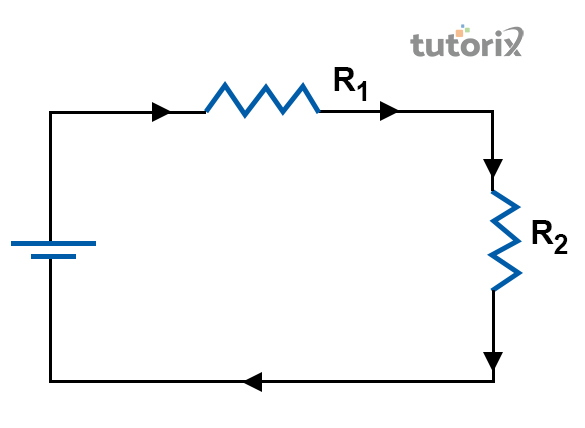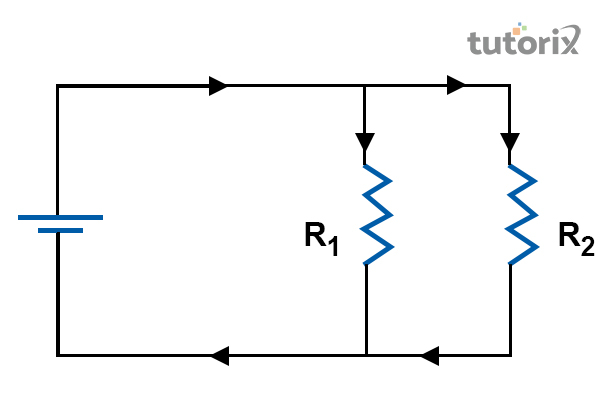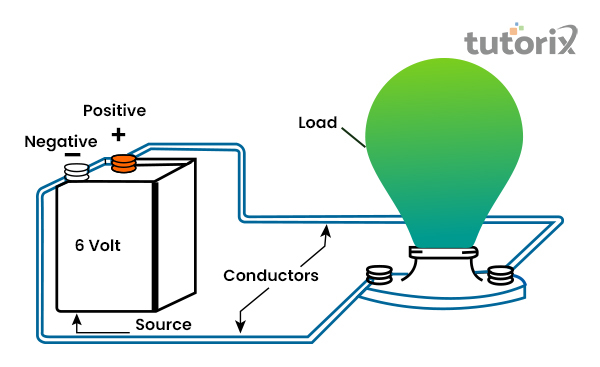# Difference Between Series and Parallel Circuits

## Introduction

The difference between these two aspects of a series circuit and a parallel circuit is that within a series circuit the same amount of current flows. Here the currents flow through the components are placed in it. Differently, it is seen that the parallel circuits shows the placing of components in different branches. It is seen that both the circuits have their own benefits and disadvantages

## Definition of Series CircuitFigure 1: Series Circuit

A Series Circuit mainly refers to a path through which currents flow through each component. In this kind of circuit, every device seems to be connected in a manner such that there is only one pathway within this circuit. It is seen that through this pathway charge can transverse the external circuit. Another important factor here is that every charge which is passing through the loop of the external circuit will also pass through each resistor in a consecutive fashion (Chaibi et al. 2018).

Therefore, in simple terms, it is said that a series circuit is a single pathway through which electricity can flow. There are many parts of a series circuit such as devices, wires, and power source and they are connected to one pathway. The devices here have no branches and they are connected along with the pathway. The main important factor here is that current flows through one branch to another.

## Definition of Parallel CircuitFigure 2: Parallel Circuit

Parallel circuit is a kind of circuit in which current passes through one or two branches. It also connects the parts at the same time before it combines again. It is seen that within a parallel circuit the potential difference, or the voltage remains the same (Linquip, 2022). In this part, it is also important to note that currently varies from one component to another. Therefore, it is seen that a parallel circuit contains multiple pathways or branches. It has different functions in this factor in this part of the application field

### Differences between Series and Parallel Circuit

Sl no Series Circuit Parallel Circuit
1. A circuit is called a series circuit when the flow of current remains the same in all components (Javatpoint, 2022).A circuit is called a series circuit when the flow of current remains the same in all components (Javatpoint, 2022). Parallel circuit refers to the circuit having two or more than two paths.
2. In this circuit all the components are arranged in a single line In this circuit, components are arranged parallel to each other (Rodrigues et al. 2020).
3. In a series circuit, $\mathrm{R\:=\:R1+R2+R3}$ In a parallel circuit, $\mathrm{R\:=\:1/R1\:+\:1/R2\:+\:1/R3}$
4. In the case of this circuit, if V is total voltage then the equation will be $\mathrm{V1+V2+V3}$. In a parallel circuit, if V is total voltage then the equation will be $\mathrm{V1=V2=V3}$.
5. The fault at a point of the circuit can break the total circuit. It does not affect the total circuit.

Table 1: Differences between Series and Parallel Circuit

Series circuit is a kind of electric circuit and the circuit have both advantages and disadvantages of this factor. Among the different advantages, one is that this kind of circuit does not heat easily.

The advantage of this circuit is that it does not catch fire easily when comes to contact with any dry or flammable object.

Another advantage of this factor is that current in all parts of a series circuit remains constant. Among the disadvantages, one common disadvantage of this factor is that when one component of the series circuit fails, then all the other components will also fail. This factor happens because the circuit has been broken. It is seen that when the components increase in the circuit, the resistance of the circuit will also become greater.

### What is an electric circuit?Figure 3: Electric Circuit

An electric circuit refers to the closed path through which electric current can flow. The construction of an electric circuit needs some major components like a resistor, battery, wire, bulb, and key (Electrical4u, 2022).

They are connected in different ways for the construction of this circuit. It is the path for the electricity to pass between two terminals of the electric cell.

## Conclusion

It s seen that a circuit can be said to as a series circuit when the flow of current remains the same within the component of the circuit. In the series circuit, current has only one path. Parallel circuits on the other hand refer to the circuit with two or more than two oaths for the passing of current. In this kind of circuit, all the components have the same voltage.

### FreFAQs

Q1. What is the concept of a parallel connection?

Parallel connection is considered a part of the electric circuit. In this circuit, the beginning of all the electrical components is connected at the same point. The important fact here is that all the component’s endpoint is connected to a particular point.

Q2. What is a series connection?

A series connection is happened within an electric circuit. In this part, the elements of a circuit are connected to each other. It means when one element is going to end, then another element starts to connect. It is seen that the current will not divide into a circuit.

Q3. What are the benefits of a parallel circuit?

The most common advantage or benefit of a parallel circuit is that voltage within this circuit remains the same in every part. It helps in productivity and here if one part is detached, it does not harm the work process.

Q4. What are the benefits of a series circuit?

There are different benefits of a series circuit and it says that the plan is straightforward. The advantage of this factor of that this circuit does not overheat rapidly. The benefit of this factor is that it conveys a similar current all through the circuit.